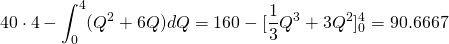# Solution assignment 03 Area and volume

### Assignment 3

Calculate the area which is enclosed by the lines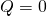and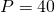and the graph of the quadratic function. This assignment is relevant for the calculation of the Producer Surplus (see Bradley T. en Patton P., Essential Mathematics for Economics and Business, 2e ed., John Wiley, p. 418).

### Solution

The intersection point of the graph of the quadratic function and the lineis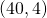. Thus the area is equal to the area of the rectangle through the points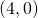and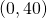minus the area enclosed by the graph and the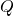-axis, betweenand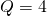: